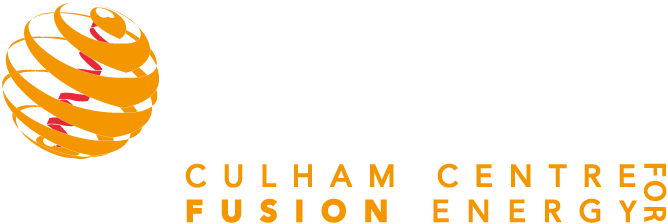# Multi-scale model for stresses, strains and swelling of reactor components under irradiation

## Multi-scale model for stresses, strains and swelling of reactor components under irradiation

Multi-scale model for stresses, strains and swelling of reactor components under irradiation 150 150 UKAEA Opendata
UKAEA-CCFE-PR(18)49

# Multi-scale model for stresses, strains and swelling of reactor components under irradiationPredicting strains, stresses and swelling in power plant components exposed to irradiation directly from the observed or computed defect and dislocation microstructure is a fundamental problem of fusion power plant design that has so far eluded a practical solution. We develop a model, free from parameters not accessible to direct evaluation or observation, that is able to provide estimates for irradiation-induced stresses and strains on a macroscopic scale, using information about the distribution of radiation defects in the microstructure. The model exploits the fact that elasticity equations involve no characteristic spatial scale, and hence admit a mathematical treatment that is an extension to that developed for the evaluation of elastic fields of defects on the nanoscale. In the analysis given below we use, as input, the radiation defect structure data derived from ab initio density functional calculations and large-scale molecular dynamics simulations of high-energy collision cascades. We show that strains, stresses and swelling can be evaluated using either integral equations, where the source functions are de ned by the density of relaxation volumes of defects, or they can be computed from heterogeneous partial differential equations for the components of the stress tensor, where the density of body forces is proportional to the gradient of the density of relaxation volumes. We perform a case study where strains and stresses are evaluated analytically and exactly, and develop a general finite-element implementation of the method, applicable to a broad range of predictive simulations of strains and stresses induced by irradiation in materials and components of any geometry in a nuclear power plant.

Collection:
Journals
Journal:
Nuclear Fusion
Publisher:
IOP
Published date:
14/09/2018